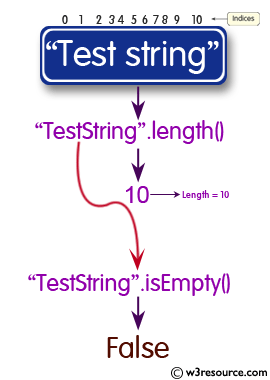# Java String: isEmpty() Method

## public String isEmpty()

The isEmpty() method is used to check if length() is 0 for a given string.

Java Platform: Java SE 8

Syntax:

`isEmpty()`

Return Value: true if length() is 0, otherwise false

Return Value Type: boolean

Pictorial presentation of Java String isEmpty() MethodExample: Java String isEmpty() Method

The following example shows the usage of java String() method.

``````import java.lang.*;

public class StringExample {

public static void main(String[] args) {

String str1 = "javaexercises";
System.out.println();
// prints length of string
System.out.println("Length of string = " + str1.length());

// checks if the string is empty or not
System.out.println("Is this string empty? = " + str1.isEmpty());
System.out.println();
}
}
```
```

Output:

```Length of string = 13
Is this string empty? = false
```

Java Code Editor:

Previous:intern Method
Next:join Method

﻿